# Test: Trigonometry- 2

## 10 Questions MCQ Test Quantitative Aptitude (Quant) | Test: Trigonometry- 2

Description
Attempt Test: Trigonometry- 2 | 10 questions in 10 minutes | Mock test for SSC preparation | Free important questions MCQ to study Quantitative Aptitude (Quant) for SSC Exam | Download free PDF with solutions
QUESTION: 1

### Practice Quiz or MCQ (Multiple Choice Questions) with solutions are available for Practice, which would help you prepare for "Trigonometry" under Quantitative Aptitude. You can practice these practice quizzes as per your speed and improvise the topic. The same topic is covered under various competitive examinations like - CAT, GMAT, Bank PO, SSC and other competitive examinations.   Q. 3sinx + 4cosx + r is always greater than or equal to 0. What is the smallest value ‘r’ can to take?

Solution: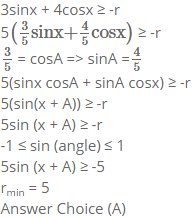QUESTION: 2

### Sin2014x + Cos2014x = 1, x in the range of [-5π, 5π], how many values can x take?

Solution:

We know that Sin2x + Cos2x = 1 for all values of x.
If Sin x or Cos x is equal to –1 or 1, then Sin2014x + Cos2014x will be equal to 1.

Sin x is equal to –1 or 1 when x = –4.5π or –3.5π or –2.5π or –1.5π or –0.5π or 0.5π or 1.5π or 2.5π or 3.5π or 4.5π.
Cosx is equal to –1 or 1 when x = –5π or –4π or –3π or –2π or –π or 0 or π or 2π or 3π or 4π or 5π.

For all other values of x, Sin2014 x will be strictly lesser than Sin2x.
For all other values of x, Cos2014 x will be strictly lesser than Cos2x.

We know that Sin2x + Cos2x is equal to 1. Hence, Sin2014x + Cos2014x will never be equal to 1 for all other values of x. Thus there are 21 values.

QUESTION: 3

### Consider a regular hexagon ABCDEF. There are towers placed at B and D. The angle of elevation from A to the tower at B is 30 degrees, and to the top of the tower at D is 45 degrees. What is the ratio of the heights of towers at B and D?

Solution:

Let the hexagon ABCDEF be of side ‘a’. Line AD = 2a. Let towers at B and D be B’B and D’D respectively.
From the given data we know that ∠B´AB = 30° and ∠D´AB = 45°. Keep in mind that the Towers B’B and D´D are not in the same plane as the hexagon.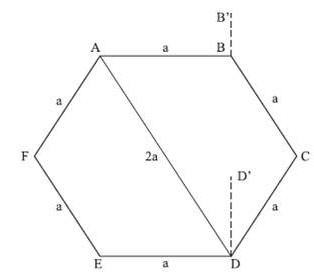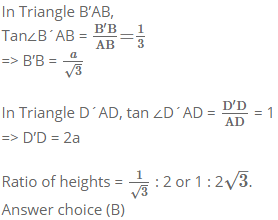QUESTION: 4

Find the maximum and minimum value of 8 cos A + 15 sin A + 15

Solution: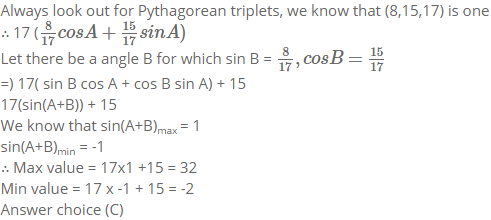QUESTION: 5

If cos A + cos2 A = 1 and a sin12 A + b sin10 A + c sin8 A + d sin6 A - 1 = 0. Find the value of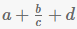Solution:

Given,
Cos A = 1- Cos2A
=) Cos A = Sin2 A
=) Cos2A = Sin4A
=) 1 – Sin2 A = Sin4 A
=) 1 = Sin4 A + Sin2 A
=) 13 = (Sin4A + Sin2A)3
=) 1 = Sin12 A + Sin6A + 3Sin8 A + 3Sin10 A
=) Sin12 A + Sin6A + 3Sin8 A + 3Sin10 A – 1 = 0
On comparing,
a = 1, b = 3 , c = 3 , d = 1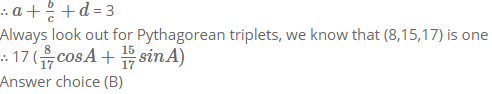QUESTION: 6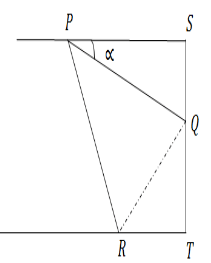In the above figure, the sheet of width W is folded along PQ such that R overlaps S Length of PQ can be written as :-

Solution:

If you are quick at observing , this question can be solved just by looking at the options as ,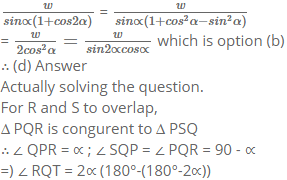We can draw the figure as :-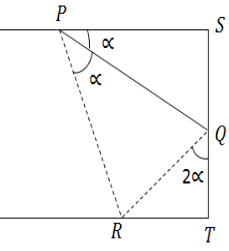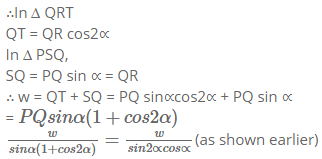QUESTION: 7

Find the value of :- (log sin 1° + log sin 2° ………..+ log sin 89°) + (log tan 1° + log tan 2° + ……… + log tan 89°) - (log cos 1° + log cos 2° + ……… + log cos 89°)

Solution:

Writing the equation as :-

(log sin 1° - log cos 89°) + (log sin 2° - log cos 88°) + (log sin 3° - log cos 87°)………… + log tan 1°. log tan 89° + log tan 2°. log tan 88° + …….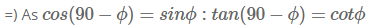=) (log sin 1° - log sin 1°) +(log sin 2° - log sin 2°)+……..+ log tan 1°cot 1° + log tan 2°cot 2°

=) log 1 = 0

QUESTION: 8

Ram and Shyam are 10 km apart. They both see a hot air balloon passing in the sky making an angle of 60° and 30° respectively. What is the height at which the balloon could be flying?

Solution: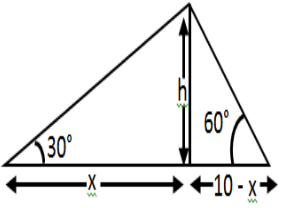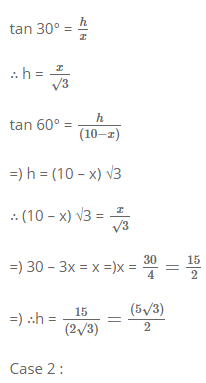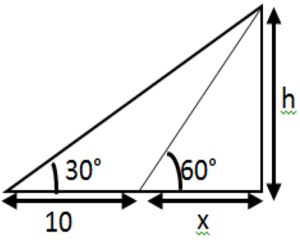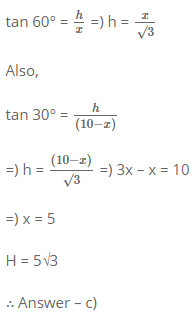QUESTION: 9

A man standing on top of a tower sees a car coming towards the tower. If it takes 20 minutes for the angle of depression to change from 30° to 60°, what is the time remaining for the car to reach the tower?

Solution: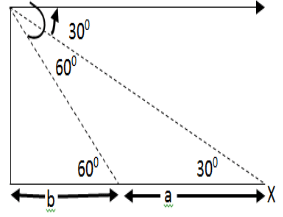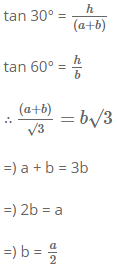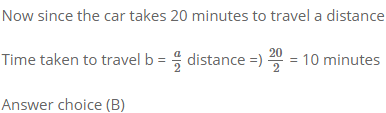QUESTION: 10

A right angled triangle has a height ‘p’, base ‘b’ and hypotenuse ‘h’. Which of the following value can h2 not take, given that p and b are positive integers?

Solution:

We know that,
h2 = p2 + b2 Given, p and b are positive integer, so h2 will be sum of two perfect squares. We see
a) 72 + 52 = 74
b) 62 + 42 = 52
c) 32 + 22 = 13
d) Can’t be expressed as a sum of two perfect squares
The question is "Which of the following value can h2 not take, given that p and b are positive integers? "Use Code STAYHOME200 and get INR 200 additional OFF Use Coupon Code